# RRB ALP 2018 Practice Test Papers | Reasoning Questions (Day-40)

Dear Aspirants, Here we have given the Important RRB ALP & Technicians Exam 2018 Practice Test Papers. Candidates those who are preparing for RRB ALP 2018 can practice these Reasoning Questions to get more confidence to Crack RRB 2018 Examination.

[WpProQuiz 2175]

Click “Start Quiz” to attend these Questions and view Solutions

1. If ‘SYNDICATE’ is written as ‘SYTENDCAI then how can ‘PSYCHOTIC’ be written?

(a) PSICYOCTH

(b) PSICYCOTH

(c) PSYICTCOH

(d) PSYCOHTCI

1. Select the missing number from the given responses.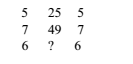(a) 38

(b) 40

(c) 36

(d) 35

1. A cyclist goes 30 km to North and then turning East he goes 40 km. Again he turns to his right and goes 20 km. After this, he turns to his right and goes 40 km. How far is he from his starting point?

(a) 25 km

(b) 40 km

(c) 6 km

(d) 10 km

1. If ‘+’ denotes ÷, ‘–’ denotes ×, ‘×’ denotes – and ‘÷’ denotes +, then 35 + 7 – 5 ÷ 5 × 6 =?

(a) 20

(b) 14

(c) 36

(d) 24

1. In the following number series a wrong number is given. Find out the wrong number.

318 158 76 38 18 8 3

(a) 38

(b) 18

(c) 158

(d) 76

DIRECTIONS (Qs. 6-7): In questions below, a series is given with one term missing. Choose the correct alternative from the given ones that will complete the series.

1. FAG, GAF, HAI, IAH, _______

(a) JAK

(b) HAK

(c) JAI

(d) HAL

1. 3, 6, 9, 15, 24, 39, 63,?

(a) 100

(b) 87

(c) 102

(d) 99

DIRECTIONS (Qs. 8 &9): Select the related letter/word/ number from the given alternatives.

1. Symphony: Composer:: Painter:?

(a) Fresco

(b) Colours

(c) Art

(d) Leonardo

1. 27: 125:: 64:?

(a) 162

(b) 216

(c) 273

(d) 517

1. 1 Unscramble the following letters to frame a meaningful word. Then find out the correct numerical position of the letters.

O T Y S R H I

1 2 3 4 5 6 7

(a) 6241375

(b) 6452173

(c) 6347125

(d) 6742153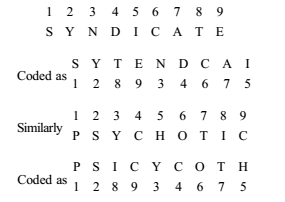The second column number is the product of first and third column

25 = 5 × 5

49 = 7 × 7

36 = 6 × 6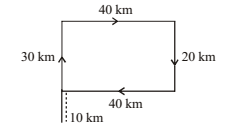35 ÷ 7 × 5 + 5 – 6 = 5 × 5 + 5 – 6

=25 + 5 – 6

=30 – 6 = 24

The series is ÷ 2 – 1 in each term.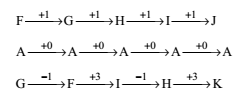3 + 3 = 6

6 + 3 = 9

9 + 6 = 15

15 + 9 = 24

24 + 15 = 39

39 + 24 = 63

63 + 39 = 102

Fresco is an art of painting that is done on freshly spread moist lime plaster.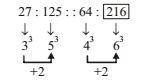H I S T O R Y

6 7 4 2 1 5 3